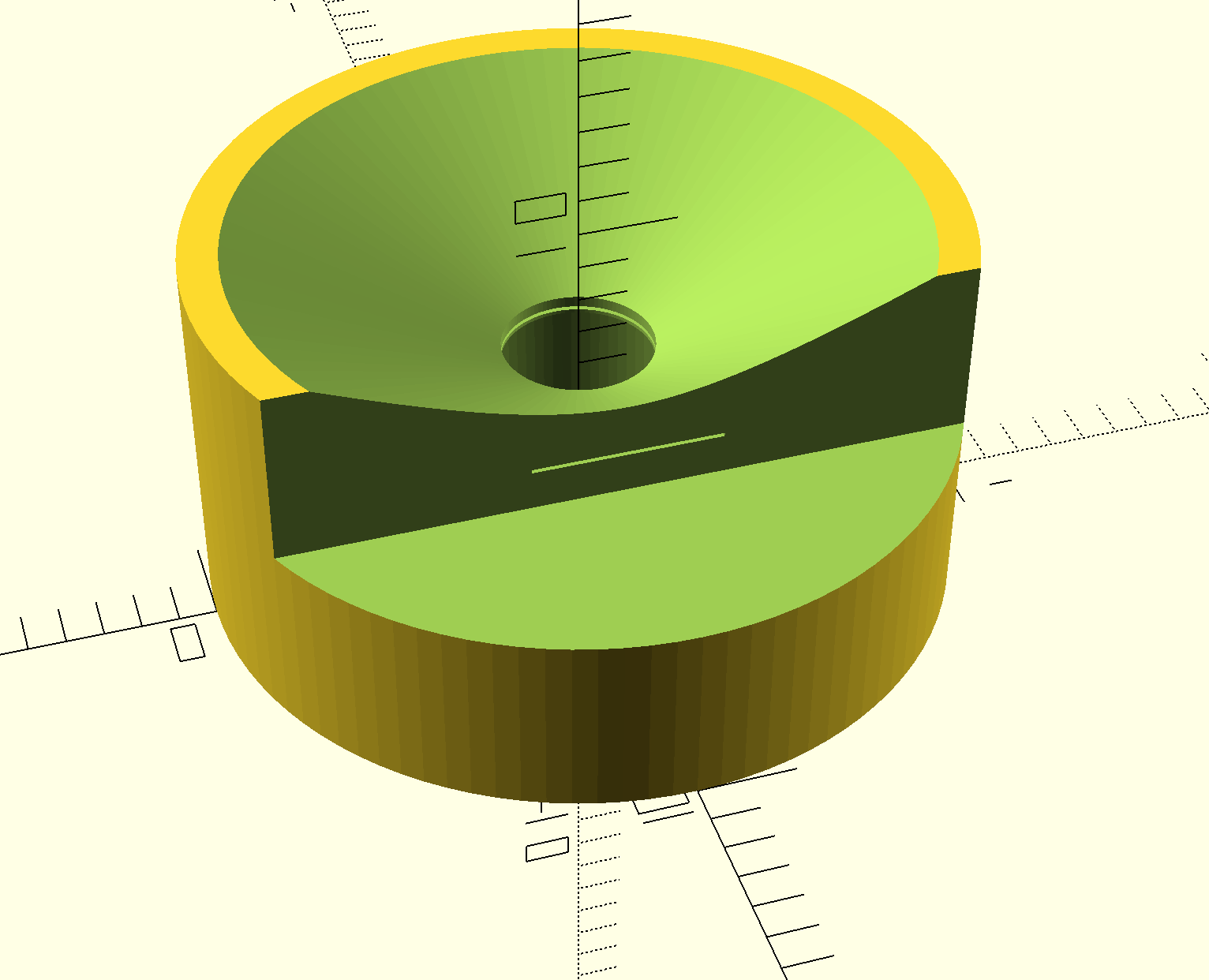# Modeling a part in OpenSCAD

Today, Chris introduced me to an open source alternative to SolidWorks for parametric modeling, OpenSCAD. It’s a bit different in that everything is defined programatically, but that has its advantages too. I was able to throw together a little camera/filter bracket without too much trouble at all.

``````module sensor_filter_bracket(module_height = 5,
total_height = 8,
filter_height = 0.1,
filter_width = 5) {

difference() {
// This is the main cylinder
cylinder(h=total_height, r=outer_radius, center=false, \$fn=100);

// Remove a cutout for the sensor module
translate([0, 0, -0.01]) {
cylinder(h=module_height + 0.01, r=inner_radius, center=false, \$fn=100);
};

translate([0, 0, module_height-0.01]) {
cylinder(h=total_height - module_height + 0.02, r=2, center=false, \$fn=100);
}

// Remove a cut out for the filter
translate([-filter_width/2, (-inner_radius-0.1), module_height+0.1]) {
};

// Remove a wider cutout from the top for imaging
translate([0, 0, module_height + 0.5]) {
cylinder(h=(total_height - module_height - 0.48), r1=(2), r2=(outer_radius - 1), center=false, \$fn=100);
};

// Remove a wider cutout from the side for filter insertion and removal
cube([outer_radius*2, outer_radius, (total_height - module_height + 1.1)]);
}
}
}

sensor_filter_bracket(module_height = 6.15,
total_height = 10,